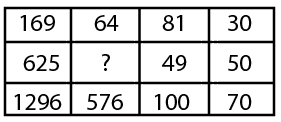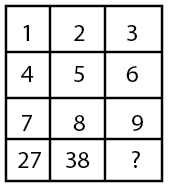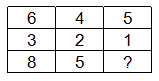# SSC Number in Grid Questions with Answers

#### Number in Grid

An excellent collection of SSC Number in grid questions and answers with detailed explanations for competitive exams. Given below are some of the most repeated practice questions on Reasoning Number in grid for SSC CGL, CHSL, JE, GD constable, Stenographer, MTS, and CPO exams. Go through this very important online quiz based on model and previous year asked questions from SSC Number in grid with solutions to ace the exam.

### Take a free SSC CHSL Mock

Thousands of students have taken Cracku's Free SSC CHSL Mock.

Instructions

For the following questions answer them individually

Question 1

Select the missing number from the given responses

Question 2

Select the missing number from the given responses.

Question 3

Select the missing number from the given responses ?

Question 4

Select the missing numbers from the given alternatives

Question 5

Select the missing number from the given responses

Question 6

Select the missing from the given responses

Question 7

Select the missing number from the given responses:

Question 8

Select the missing number from the given responses.

Question 9

Select the missing number from the given responses

Question 10

Select the missing numbers from the given alternatives

Question 11

Select the missing number from the given responses ?

Question 12

Select the missing number from the given responses

Question 13

Select the missing number from the given responses ?

Question 14

Select the missing number from given responses

Question 15

In the question, selection the missing number from the given alternatives.

Question 16

Select the missing numbers from the given alternatives

Question 17

Select the missing number from the given responses

Question 18

Select the missing numbers from the given alternatives

Instructions

In each of the following questions, select the missing number from the given responses.

Question 19Instructions

For the following questions answer them individually

Question 20

Select the missing number from the given responses:

Question 21

Select the missing number from the given responses

Instructions

In each of the following questions, select the missing number from the given responses.

Question 22Instructions

For the following questions answer them individually

Question 23

Select the missing number from the given responses.Question 24

Select the missing numbers from the given alternatives

Question 25

Select the missing number from the given responses.